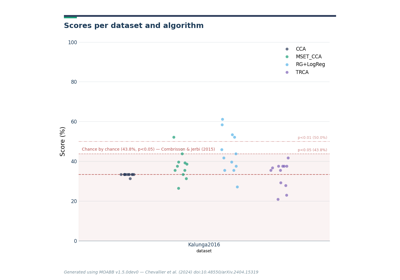SSVEP paradigm with multiple narrow bandpass filters, centered around the frequencies of considered events. Metric is ‘roc-auc’ if 2 classes and ‘accuracy’ if more.

Parameters
• filters (list of list | None (default None)) – If None, bandpass set around freqs of events with [f_n-0.5, f_n+0.5]

• events (List of str,) – List of stimulation frequencies. If None, use all stimulus found in the dataset.

• n_classes (int or None (default 2)) – Number of classes each dataset must have. All dataset classes if None

• tmin (float (default 0.0)) – Start time (in second) of the epoch, relative to the dataset specific task interval e.g. tmin = 1 would mean the epoch will start 1 second after the begining of the task as defined by the dataset.

• tmax (float | None, (default None)) – End time (in second) of the epoch, relative to the begining of the dataset specific task interval. tmax = 5 would mean the epoch will end 5 second after the begining of the task as defined in the dataset. If None, use the dataset value.

• baseline (None | tuple of length 2) – The time interval to consider as “baseline” when applying baseline correction. If None, do not apply baseline correction. If a tuple (a, b), the interval is between a and b (in seconds), including the endpoints. Correction is applied by computing the mean of the baseline period and subtracting it from the data (see mne.Epochs)

• channels (list of str | None (default None)) – List of channel to select. If None, use all EEG channels available in the dataset.

• resample (float | None (default None)) – If not None, resample the eeg data with the sampling rate provided.

Attributes
datasets

Property that define the list of compatible datasets

scoring

Property that defines scoring metric (e.g.

Methods

 get_data(dataset[, subjects, return_epochs]) Return the data for a list of subject. is_valid(dataset) Verify the dataset is compatible with the paradigm. prepare_process(dataset) Prepare processing of raw files process_raw(raw, dataset[, return_epochs]) Process one raw data file.
 used_events